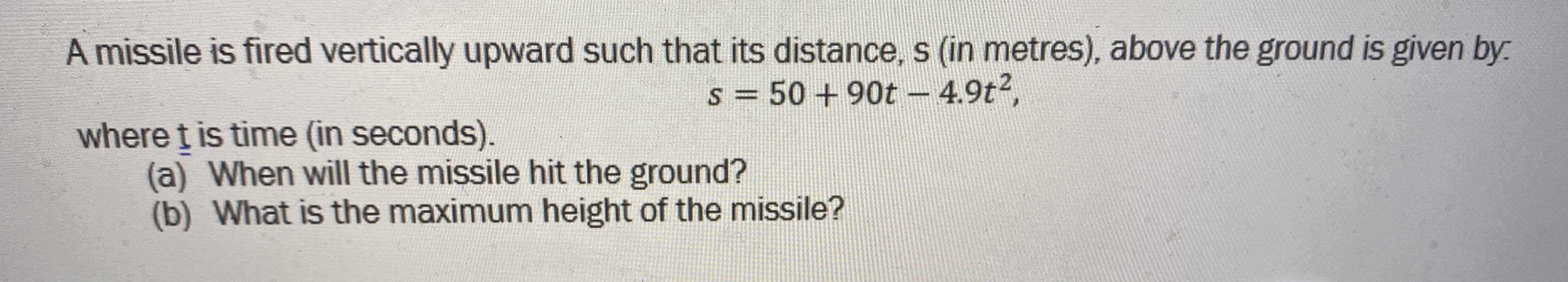### ¿Todavía tienes preguntas de matemáticas?

Pregunte a nuestros tutores expertos
Algebra
PreguntaA missile is fired vertically upward such that its distance, $$s$$ (in metres), above the ground is given by.

$$s = 50 + 90 t - 4.9 t ^ { 2 } ,$$

where t is time (in seconds). (a) When will the missile hit the ground? (b) What is the maximum height of the missile?

a) When the missile hits the ground, s = 0

-4.9t^2 + 90 t + 50 = 0

t = ( -90 $$\pm$$$$\sqrt{90^2 - 4 (- 4.9) (50)}$$  ) / 2 * -4.9

On solving the above, we get t = 18.907 seconds

b) For max. height, differentiate 's' w.r.t t and equate to 0

$$\dot{s}$$ = -9.8 t + 90 = 0

t = 90 /9.8 = 9.183

Max height occurs at t = 9.183 seconds

So s at t = 9.183

Max height = s(9.183)= 50 + 90  9.183 -  4.9 (9.183^2)

Max. Height = 463.325 metres

Solución
View full explanation on CameraMath App.